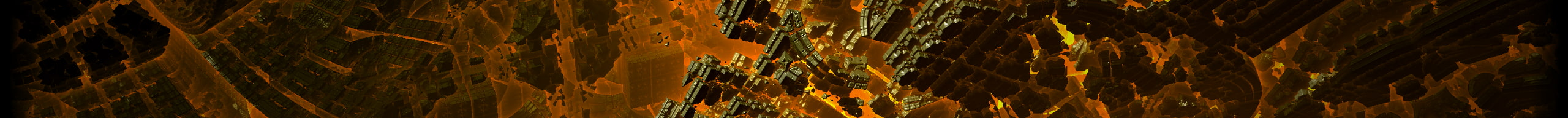# 29a.chby Jonas Wagner# Demonstrating evolution in 999b

By

When I saw the JS1K demo contest I knew I had to enter. I first tried to do some GFX demo, but I was lacking an impressive idea. So when I tried to sleep last night at 2 in the morning I had an idea which I needed to implement: Demonstrating evolution by natural selection in 999b/666b gzipped:

```x = ('var_a=Math,b=a.random,c=document.g'+
'etElementById("c"),e=c.getContext("2d")'+
',f=[],g=b()*		 16777216<<0,h,i'+
',j,k,l=(g		  &16711680)>>16'+
',m=(g&65		    280)>>8,n=g&'+
'255,o,p		    ;c.width=320'+
'=10;for /* */	      (p=   0;p<64;p++)f'+
'.push(b(		    )*16777216<<'+
'0);func		    tion_q(d){fo'+
'r(d=d.t oStr		  ing(16);d.leng'+
'th<6;)			d = " 0"+d;retur'+
'n"#"+		      d } d ocument.body'+
'.sty		     l e .b a c kground='+
'q(g);		    setInterval(function'+
'(){e.		 c l e a r R e c t ( 0 ,'+
'0,320,		3 2 0 ) ; f o r ( p = 0 '+
';p<64;	    p + + ) { h = f [ p ] ; i = '+
'l-((h&	  1 6 7 1 1 6 8 0 ) > > 1 6 ) ;j'+
"=m-((h&65 2 8 0 ) > > 8 ) ; k = n - ( h"+
'&255);o=a.sqrt(i*i+j*j+k*k)/443;if(b()<'+
"o)h=f[p]=(f[b()*64<<0]&16773120|f[b()*6"+
'4<<0]&4095)^1<<(b()*24<<0);e.fillStyle='+
"q(h);e.fillRect(1+p%8<<5,1+p/8<<5,32,32"+
/\s/g,"").replace(/_/g,' ');eval(x);
/*evolution in 999b by 29a.ch**/
```

+1 if you recognize that guy.

# Demo

It's basically a few cells colored cells. The selection criteria is the color difference between the cell and the background. So cells that stand out have a higher chance of getting eaten.

Click me

# How it works

You can see the full unminimized sourcecode here evolution.js I'll only explain the meaty stuff.

```env = random()*(1<<24)<<0,
...
population.push(random()*(1<<24)<<0)
```

A random environment and population is initialized. The shift by 0 is a short way to round a number. Both the environment and the genes of the population are random 24 bit integers, that can be viewed as rgb colors.

```subject=population[i];
r = r_-((subject&0xff0000)>>16);
g = g_-((subject&0xff00)>>8);
b = b_-(subject&0xff);
distance=M.sqrt(r*r+g*g+b*b)/443;
if(random()<distance){
```

The fitness for survival is determined by measuring the distance between the color of the individual and the environment. The lower the distance, the higher the chance for survival.

```subject = population[i] = (
// breed
((population[random()*popsize<<0]&0xfff000)|
(population[random()*popsize<<0]&0xfff))
// Mutate
^(1<<(random()*24<<0))
);
```

When an individual dies, a new one is bred by sexual reproduction (parthenogenesis and necrophilia are possible as well). The new individual then gets one random mutation. That's pretty much all there is to it.